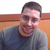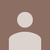0

# Combining Contours

Nathan Smela 6 years ago updated by ไอยดา สุรีวงค์ 2 years ago

Heya All,

I want the total contour of a mesh as if viewing from top-down on the z axis.  I grab a contour every 1 mm on the z axis, and then move all points to 0 z-axis.  Is there a method to contour around these points?

{
//abort if the mesh is invalid
if (mesh == null || mesh.TriangleIndices.Count < 3)
return;

var bounds = mesh.Bounds;

List<Point3D> pointmap = new List<Point3D>(); //collects all the points from contouring

//setting the contour plane

Point3D plane = new Point3D();
Vector3D normal = new Vector3D(0,0,1);

//cycles through the mesh, grabbing a contour at each z interval
//combines all on 0 on the z-axis
for (double z = 0.1; z < bounds.SizeZ; z += 0.1)
{
plane = new Point3D(0, 0, z);
var segments = MeshGeometryHelper.GetContourSegments(mesh, plane, normal).ToList(); //calculates the contour

foreach (var point in segments)
}

//convert pointmap to a contour

//done converting contour

var mb = new MeshBuilder(true, false);
mb.CreateNormals = false;